# Flipping a MATRIX in many ways (Directions) in MATLAB: Column flip, Row flip & Diagonal flip

Flipping a matrix? What would be the use of it?
Well the first use of it that comes to my mind is applicable on images. Sometimes we need to flip it, upside down, side ways, with the main diagonal or the other diagonal, since at the basic level image is a matrix we can apply the transformations of a matrix to an image also, but that depends on our application.

Take a randomly generated matrix 'x', such that it can be seen as,

>> x=rand(6,6)
x =
0.8147    0.2785    0.9572    0.7922    0.6787    0.7060
0.9058    0.5469    0.4854    0.9595    0.7577    0.0318
0.1270    0.9575    0.8003    0.6557    0.7431    0.2769
0.9134    0.9649    0.1419    0.0357    0.3922    0.0462
0.6324    0.1576    0.4218    0.8491    0.6555    0.0971
0.0975    0.9706    0.9157    0.9340    0.1712    0.8235

If we want to flip the matrix column wise, i.e, the first & the last column will be exchanged, we have to write the MATLAB command as:
>> x(:,end:-1:1) = x
Which will result in,
x =
0.7060    0.6787    0.7922    0.9572    0.2785    0.8147
0.0318    0.7577    0.9595    0.4854    0.5469    0.9058
0.2769    0.7431    0.6557    0.8003    0.9575    0.1270
0.0462    0.3922    0.0357    0.1419    0.9649    0.9134
0.0971    0.6555    0.8491    0.4218    0.1576    0.6324
0.8235    0.1712    0.9340    0.9157    0.9706    0.0975

Just Compare it from the original matrix, you will find that the first & the last column & all the corresponding columns are exchanged.

Again if we want to get back the original matrix, then we need to just enter the previous flipping command again,
>> x(:,end:-1:1) = xThe resultant matrix is back to original one.
x =
0.8147    0.2785    0.9572    0.7922    0.6787    0.7060
0.9058    0.5469    0.4854    0.9595    0.7577    0.0318
0.1270    0.9575    0.8003    0.6557    0.7431    0.2769
0.9134    0.9649    0.1419    0.0357    0.3922    0.0462
0.6324    0.1576    0.4218    0.8491    0.6555    0.0971
0.0975    0.9706    0.9157    0.9340    0.1712    0.8235

What if want to flip the matrix row wise?
The with a slight variation of the above command in which we have flipped the matrix column wise, will result in a flip row wise.

The command we need here is:
x(end:-1:1,:) = x
x =
compare the resultant matrix with the original one, you will find that the matrix is flipped row wise.
0.0975    0.9706    0.9157    0.9340    0.1712    0.8235
0.6324    0.1576    0.4218    0.8491    0.6555    0.0971
0.9134    0.9649    0.1419    0.0357    0.3922    0.0462
0.1270    0.9575    0.8003    0.6557    0.7431    0.2769
0.9058    0.5469    0.4854    0.9595    0.7577    0.0318
0.8147    0.2785    0.9572    0.7922    0.6787    0.7060

Again, in order to recover the matrix from the row flipped version we have to retype the command x(end:-1:1,:) = x.

NOTE: better use a temporary variable in order to preserve the original matrix.

Now its the turn of flipping the matrix through the matrix along with its 2 diagonals.

These example will work if we have a square matrix with odd number or rows/columns.

Flipping the matrix along its main diagonal.
The thing with flipping the matrix along with its main diagonal is, that its simply its transpose, for example we will generate a 5x5 random matrix & apply the flipping transformation through the transpose.

x=rand(5,5)
A 5x5 random matrix
x =
0.7572    0.0540    0.5688    0.7943    0.2630
0.7537    0.5308    0.4694    0.3112    0.6541
0.3804    0.7792    0.0119    0.5285    0.6892
0.5678    0.9340    0.3371    0.1656    0.7482
0.0759    0.1299    0.1622    0.6020    0.4505

>> x'  %flipping the matrix along with its main diagonal
ans =On comparing with the original matrix you will find this matrix flipped along its main diagonal.
0.7572    0.7537    0.3804    0.5678    0.0759
0.0540    0.5308    0.7792    0.9340    0.1299
0.5688    0.4694    0.0119    0.3371    0.1622
0.7943    0.3112    0.5285    0.1656    0.6020
0.2630    0.6541    0.6892    0.7482    0.4505

Flipping the matrix along its non main diagonal or the other diagonal.

Well thats a little bit tricky...., but here it is.... its a series of 2 commands

x=rand(5,5)
%generate a 5x5 random matrix for further operation
x =
0.0838    0.5383    0.9619    0.0844    0.9106
0.2290    0.9961    0.0046    0.3998    0.1818
0.9133    0.0782    0.7749    0.2599    0.2638
0.1524    0.4427    0.8173    0.8001    0.1455
0.8258    0.1067    0.8687    0.4314    0.1361

>> x=x'; %first generate the transpose
>> x(end:-1:1)=x %then flip it
%on comparing with the original matrix we find that following the above steps that the matrix is flipped along the other diaginal
x =
0.1361    0.1455    0.2638    0.1818    0.9106
0.4314    0.8001    0.2599    0.3998    0.0844
0.8687    0.8173    0.7749    0.0046    0.9619
0.1067    0.4427    0.0782    0.9961    0.5383
0.8258    0.1524    0.9133    0.2290    0.0838

# Pulse Code Modulation (PCM) Using MATLAB

PCM is a type of source coding.
Invented by Alec Reeves, it is the standard form of digital audio, CDs, telephony & other digital audio applications. A PCM stream is a digital representation of an analog signal, in which the magnitude of the analogue signal is sampled regularly at uniform intervals, with each sample being quantized to the nearest value within a range of digital steps.

A PCM stream has two basic properties that determine the stream's fidelity to the original analog signal: the sampling rate, which is the number of times per second that samples are taken; and the bit depth, which determines the number of possible digital values that can be used to represent each sample.PCM Modulated Sine Wave (Courtesy : Wikipedia)
In the diagram above, a sine wave (red curve) is sampled and quantized for PCM. The sine wave is sampled at regular intervals, shown as vertical lines. For each sample, one of the available values (on the y-axis) is chosen by some algorithm. This produces a fully discrete representation of the input signal (blue points) that can be easily encoded as digital data for storage or manipulation. For the sine wave example at right, we can verify that the quantized values at the sampling moments are 8, 9, 11, 13, 14, 15, 15, 15, 14, etc. Encoding these values as binary numbers would result in the following set of nibbles: 1000 (23×1+22×0+21×0+20×0=8+0+0+0=8), 1001, 1011, 1101, 1110, 1111, 1111, 1111, 1110, etc. These digital values could then be further processed or analyzed by a digital signal processor.

MATLAB Implementation (Simulation) Of Pulse Code Modulation (PCM)
clc
close all
clear all
t = 0:0.0001:20;
%sampling at niquist rate
c=input('Enter Bit Depth Of PCM Coding:');
part = -1:0.1:1;
%A quantization partition defines several contiguous, nonoverlapping ranges
%of values within the set of real numbers.
codebook = -1:0.1:1.1;
%A codebook tells the quantizer which common value to assign to inputs that
%fall into each range of the partition.
msg = cos(t);
[~,quants] = quantiz(msg,part,codebook);
%returns a vector that tells which interval each input is in
subplot(3,1,1);
plot(t,msg);
title('Message Signal');
subplot(3,1,2);
plot(t,quants);
title('Quantized Signal');
y = uencode(quants,c);
ybin=dec2bin(y,c);
%converting it to final binary form to make it transmit ready
subplot(3,1,3);
plot(t,y);
title('PCM PLOT');

Some Concepts Improtant for Coding Implementation:
Two parameters that determine a quantization: a partition and a codebook.
A quantization partition defines several contiguous, nonoverlapping ranges of values within the set of real numbers.
For example, if the partition separates the real number line into the four sets
• {x: x ≤ 0}
• {x: 0< x ≤ 1}
• {x: 1 < x ≤ 3}
• {x: 3 < x}
then you can represent the partition as the three-element vector
`partition = [0,1,3]; `
` `
A codebook tells the quantizer which common value to assign to inputs that fall into each range of the partition. Represent a codebook as a vector whose length is the same as the number of partition intervals. For example, the vector
codebook = [-1, 0.5, 2, 3]; is one possible codebook for the partition [0,1,3].
` `

NOTE: The output PCM Plot & value projection greatly depends on the bit depth you have chosen. More the bit depth used, more closely it will resemble the message signal while plotting the curve.

Sample Input & Output:

Enter Bit Depth Of PCM Coding:2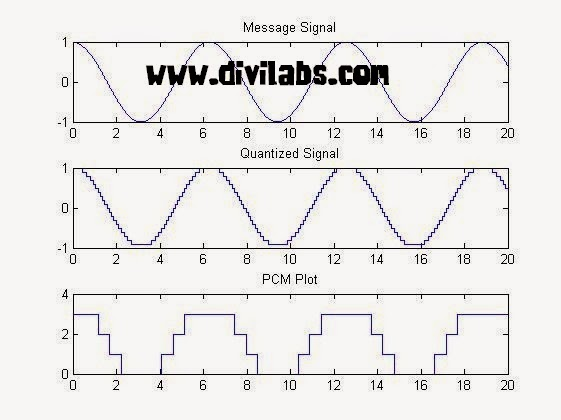PCM (Pulse Code Modulation) in MATLAB 2 bit Coding

Enter Bit Depth Of PCM Coding:8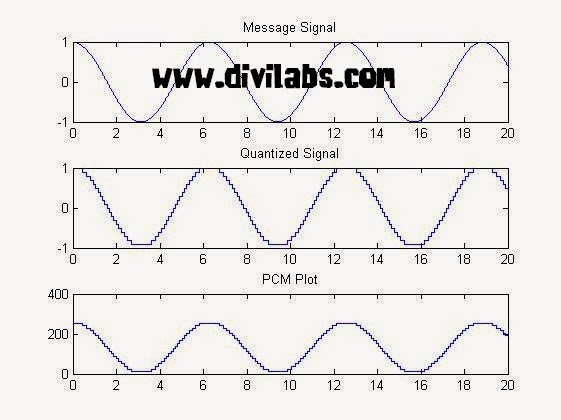PCM (Pulse Code Modulation) in MATLAB 8 bit Coding
Hence its also clear by seeing above two implementation that more the bit depth more accurately the message signal are coded. But the drawback of increasing the bit depth is, more number of bits is now to be transmitted.

# Even & Odd Parity Check, Generate & Add Parity Bit, in a Bin Sequence Using MATLAB

A parity bit is a bit added to the end of a sequence of bits (zeros or ones) that indicates whether the number of bits in the string with the value one is even or odd. Parity bits are used as the simplest form of error detecting code.

Assume, for example, that two devices are communicating with even parity, the transmitting device sends data, it counts the number of set bits in each group of bits. If the number of set bits is even, it sets the parity bit to 0; if the number of set bits is odd, it sets the parity bit to 1. In this way, every sequence have an even number of set bits. On the receiving side, the device checks to make sure that it has an even number of set bits. If it finds an odd number of set bits, the receiver knows there was an error during transmission.

NOTE:
The sender and receiver must both agree to use parity checking and to agree on whether parity is to be odd or even. If the two sides are not configured with the same parity sense, communication will be impossible.

MATLAB Code for Even Parity Check & Adding Parity Bit, in an User Dfined Bin Sequence

clc;
close all;
clear all;
x = input('Enter the bit sequence to test for Even parity: ');
t = 0;
for i = 1:length(x)
%can replace this 'for' loop just by t=sum(x)
if(x(i))
t = t + 1;
%increment by one if a bit is one
end
end

if(mod(t,2)~=0) %check if not even then attach another '1' to make the parity even
y = [x 1]; disp('Parity bit generated : 1');
else
%check if even then attach another '0' to let the parity be even
y = [x 0]; disp('Parity bit generated : 0');
end
disp('Input bit sequence:');
disp(x);
%display the input bit sequence
disp('Bit sequence with parity (Even) bit : ');
disp(y);
%display the resultant bit sequence after parity bit addition

Sample input output for  Even Parity Check & Adding Parity Bit
(1)
Enter the bit stream : [1 0 1 1 0 1 1]
Input bit stream :      1     0     1     1     0     1     1
Parity bit generated : 1
Transmitting message sequence including parity bit :     1     0     1     1     0     1     1     1

(2)
Enter the bit sequence to test for Even parity: [1 0 1 1 0 1 0]
Input bit sequence:     1     0     1     1     0     1     0
Parity bit generated : 0
Bit sequence with parity (Even) bit :     1     0     1     1     0     1     0     0

MATLAB Code for Odd Parity Check & Adding Parity Bit, in an User Dfined Bin Sequence

clc;
close all;
clear all;
x = input('Enter the bit sequence to test for Odd parity: ');
t = 0;
for i = 1:length(x)
%can replace this 'for' loop just by t=sum(x)
if(x(i))
t = t + 1;
%increment by one if a bit is one
end
end

if(mod(t,2)~=1) %check if not odd then attach another '1' to make the parity odd
y = [x 1]; disp('Parity bit generated : 1');
else %check if odd then attach another '0' to let the parity be odd
y = [x 0]; disp('Parity bit generated : 0');
end
disp('Input bit sequence:');
disp(x);
%display the input bit sequence
disp('Bit sequence with parity (Odd) bit : ');
disp(y);
%display the resultant bit sequence after parity bit addition

Sample input output for  Odd Parity Check & Adding Parity Bit
(1)
Enter the bit sequence to test for Odd parity: [1 0 1 1 0 1 0]
Input bit sequence:     1     0     1     1     0     1     0
Parity bit generated : 1
Bit sequence with parity (Odd) bit:      1     0     1     1     0     1     0     1
(2)
Enter the bit sequence to test for Odd parity: [1 0 1 1 0 1 1]
Input bit sequence:
1     0     1     1     0     1     1
Parity bit generated : 0
Bit sequence with parity (Odd) bit:
1     0     1     1     0     1     1     0

# PWM (Pulse Width Modulation) Using MATLAB

Pulse Width Modulation (PWM) is one method of reducing the perceived luminance in displays, which it achieves by cycling the backlight on and off very rapidly. This generally means that at 100% brightness a constant voltage is applied to the backlight and it is continuously lit. As you lower the brightness control the perceived luminance for the user reduces due to a number of possible controlling factors: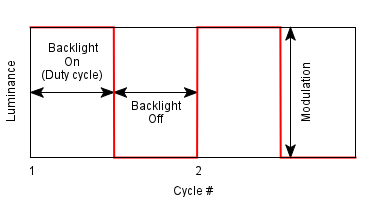How the LED LUMA depends on the pulse fed into it, (coursey: tft central)
In short PWM simply does modulation of the duty cycle in accordance with the amplitude of message signal & a comparator reference sawtooth wave. In addition to the Display use, it find use in communication systems, voltage regulators such as Switched Mode Power Supplies (SMPS) to control the power delivered to the load, LED circuits (particularly of fading Effect), Motor Speed Control etc.PWM generation process (Courtesy: Wikipedia)
MATLAB Code for PWM (Pulse Width Modulation):

fs=input('Comparator Sawtooth frequency:');
fm=input('Message frequency(Assuming it to be a sine wave):');
a=input('Enter Amplitude of Message:');

t=0:0.0001:1; %sampling rate of 10kHz

stooth=1.01*a.*sawtooth(2*pi*fs*t);
%generating a sawtooth wave
%to make the two non zero lobes of pwm not to overlap the amplitude of
%sawtooth wave must be atleast more than a bit to the message amplitude

subplot(3,1,1);
plot(t,stooth);
% plotting the sawtooth wave
title('Comparator Wave');

msg=a.*sin(2*pi*fm*t);
%generating message wave

subplot(3,1,2);
plot(t,msg);
%plotting the sine message wave
title('Message Signal');

for i=1:length(stooth)
if (msg(i)>=stooth(i))
pwm(i)=1;
%is message signal amplitude at i th sample is greater than
%sawtooth wave amplitude at i th sample
else
pwm(i)=0;
end
end

subplot(3,1,3);
plot(t,pwm,'r');
title('PWM');
axis([0 1 0 1.1]);
%to keep the pwm visible during plotting.

Sample Input:

Comparator Sawtooth frequency:10
Message frequency(Assuming it to be a sine wave):1
Enter Amplitude of Message:5

Results of MATLAB code for PWM (Pulse Width Modulation):PWM (Pulse Width Modulation) Using MATLAB

# MATLAB Based Salt & Pepper Noise Removal by 2D Median Filtering without medfilt2 MATLAB Function

## A 2-D Median filter

The 2D median filter is a sliding-window spatial filter, it replaces the central value in the window with the median of all the pixel values lying in the window. An example of median filtering of a single 3x3 window of values is shown below.

 7 3 0 4 33 5 20 4 11

in ascending order they are:
0, 3, 4, 4, 5, 7, 11, 20, 33

 * * * * 5 * * * *
Center value (previously 33) is replaced by the median of all nine values (5).
The median filter is also widely claimed to be 'edge-preserving' since it theoretically preserves step edges without blurring. However, in the presence of noise it does blur edges in images slightly.

How 2d median filter is applied on an Image Matrix, through a sliding window approach. Due to 2nd & 3rd condition we need to pad the edges.(Image illustration Courtesy, CUVT Prague)
By the above illustrations, it might be clear till now how the central element of the median filter is calculated & how the sliding window approach helps to decide & replace the original valued pixel.

Also before going to the implementation of MATLAB Based Salt & Pepper Noise Removal by 2D Median Filtering without medfilt2 MATLAB Function, I suggest you to please refer the below two articles, for having a much enhanced insight of the problem.

# Original Image:Original Image

# Grayscale Version of the original image:# Grayscale Version of the original image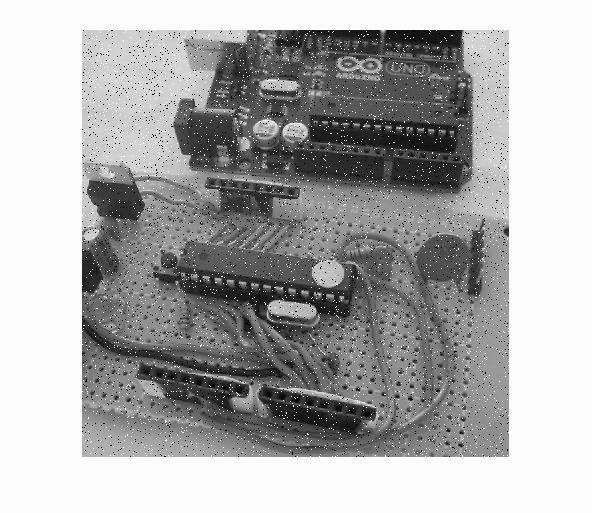Image after adding the Salt & Pepper Noise
Input:
Enter the size of kernel of median filter, (n for nxn matrix):4

Resultant Noise Removed Image:Resultant Noise Removed Image, With a kernel size 4x4

# Padding Borders of an Image with Zeros or Any Constant Value, Using MATLAB

Why Padding of borders is required While working with an Image?

When you apply a filter to pixels on the borders of an image, some of the elements of the masking block or filter kernel may not overlap actual image pixels. For example, if the kernel is 3-by-3 and you are computing the result for a pixel on the top row of the image, some of the elements of the kernel are outside the border of the image. For example see the below image,(Image illustration Courtesy, CUVT Prague)

This technique is also used by the software like MS Word, PhotoShop when we want to add a border in the image.

Padding zeros in the border of an Image: MATLAB Implementation

Suppose you are having any image;Original Grayscale image ready to be padded with zero or any other constant value
MATLAB Code for padding Zeros on the boundary of an image:

clc
clear all
close all

%i=rgb2gray(i); %Uncomment this to Convert the image to grayscale if original one is RGB
imshow(i) %Show the original image before preprocessing
p=input('Input no. of rows/columns to pad:');
%Ask the user to get the number of rows/columns to be padded
%generate an initial zero matrix with the dimension added with 2*p more.
for x=1:size(i,1)
for y=1:size(i,2)
%accessing the x+p & y+p location and storing  x,y location pixel into that
end
end

Result of above code to pad zero in an image:
Input no. of rows/columns to pad:6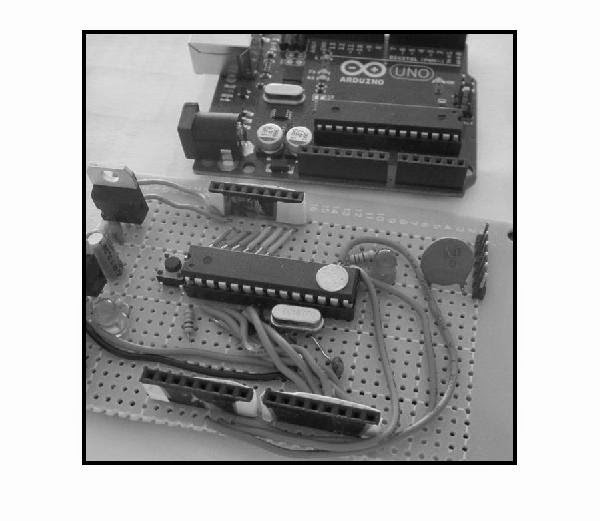Zeros padded grayscale image, Using MATLAB
Original Dimension if the image: 640x640
Resultant Dimension if the image: 652x652
A part of the resultant Image with a corner looks like:
0    0    0    0    0    0      0        0        0        0        0
0    0    0    0    0    0      0        0        0        0        0
0    0    0    0    0    0      0        0        0        0        0
0    0    0    0    0    0      0        0        0        0        0
0    0    0    0    0    0      0        0        0        0        0
0    0    0    0    0    0      0        0        0        0        0
0    0    0    0    0    0    217    217    217    217    217
0    0    0    0    0    0    217    217    217    216    216
0    0    0    0    0    0    217    217    217    216    216
0    0    0    0    0    0    218    218    217    217    216
0    0    0    0    0    0    219    218    218    217    216
0    0    0    0    0    0    217    217    217    216    216
0    0    0    0    0    0    214    214    214    214    214
0    0    0    0    0    0    212    212    212    212    212

MATLAB Code for padding Any Constant Number on the boundary of an image:
%With a little modification of the above code we can achieve that
%The new code will be like
clc
clear all
close all

%i=rgb2gray(i); %Uncomment this to Convert the image to grayscale if original one is RGB
imshow(i) %Show the original image before preprocessing
p=input('Input no. of rows/columns to pad:');
%Ask the user to get the number of rows/columns to be padded
v=input('The value of the constant to be padded:');
%generate an initial zero matrix with the dimension added with 2*p more.
for x=1:size(i,1)
for y=1:size(i,2)
%accessing the x+p & y+p location and storing  x,y location pixel into that
end
end

Now after an execution of the code:
Input no. of rows/columns to pad:4
Value of the costant? 4

Our Padded Image matrix will look like:
4    4    4    4      4        4        4        4        4
4    4    4    4      4        4        4        4        4
4    4    4    4      4        4        4        4        4
4    4    4    4      4        4        4        4        4
4    4    4    4    217    217    217    217    217
4    4    4    4    217    217    217    216    216
4    4    4    4    217    217    217    216    216
4    4    4    4    218    218    217    217    216
4    4    4    4    219    218    218    217    216

# Adding Salt & Pepper Noise To An Image Using MATLAB : Using & Without Using IMNOISE Function

What is Salt & Pepper Noise?
Its an impulsive noise, which presents itself as sparsely occurring white and black pixels over an image. Its a data drop-out noise (commonly known as intensity spikes or salt and pepper noise). The noise here is caused generally by errors in the data transmission. Remember the snow like video seen on old CRT television sets, when the channel reception fails, its like the same. The corrupted pixels either set to the maximum value or to a min value (generally 240-255 for salt & 0-8 for pepper noise). Because of their color & appearance in the image they are named like that.
In worst case of transmission, where the data becomes completely corrupted, single pixels are set alternatively to zero or to the maximum value, giving the image a 'salt and pepper' like appearance. The noise is usually quantified by the percentage of pixels which are corrupted.

How to add Salt & Pepper Noise in Your Image Using MATLAB
The original image we have used is this:Method 1: By using Inbuilt "imnoise" function of MATLAB

Syntax to use 'imnoise' is,
# X = imnoise(Image, 'salt & pepper', percentage_distortion)
Above function adds "salt and pepper" noise to an image named Image, where percentage_distortion is the noise density.
# This affects approximately percentage_distortion*numel(I) pixels. The default for percentage_distortion is 0.05.

Usage:
new_i=imnoise(i,'salt & pepper', 0.05); %noise added in this step for a 5% corrupted pixels
imshow(new_i)

Results:NOTE: The function imnoise will work same for Grayscale & RGB Color image same, without any modification.

Method 1: With come logic & mathematics using MATLAB

Step 1: Select a value of Black & White Pixels of your image; For Black Choose a value of 0-8 & for a white one choose a value of 240-255. (These value will give you the appearance of salt and pepper spread over the image) . The default value in many cases are assumed to be 0 & 255 for Black & White pixels respectively, that particularly happens in high contrast images.

Step 2: Generate a random pixel to make it corrupt.

Step 3: Apply the logic to change the pixel value.

MATLAB Code Implementation of Adding Salt & Pepper noise without using imnoise is:

b=4;w=251;  %assuming our black pixel is having a value of '4' & the white one is '251'
img_with_noise= i;
%preserving the original image 'i' & operating on a new variable
[m,n]=size(i); %getting the size of image
x = randint(m,n,[0,255]);  % **See below note
%generating a random matrix of size mxn which is the size of image
%whose value of each element is randomly distributed
%over a range of 0 to 255
img_with_noise(x <= b) = 0;
%setting that pixel value '0' whose pixel value is below or equal '4'
%this step will add the pepper noise in the image

img_with_noise(x >=w) = 255;
%setting that pixel value to '255' whose pixel value is >= '251'
%this step will add the salt noise in the image
imshow(img_with_noise)%Show the corrupted image

Result of application of Above MATLAB code is:NOTE: * To make this applicable for even rgb images, use the commands to extract the image color planes & apply on them (For more reference Visit: Extracting Individual RGB Image Planes)

**The  % of the corrupted pixels which are either more biased towards salt noise or pepper noise can be controlled by the statement, x = randint(m,n,[0,255]); For making the resultant image more salty like, use any value less than '255', or to make it more pepperish use any value above '0'

*** The percentage of the corrupted pixels which are euqlly pepper & salt can be done by taking the value of 'b' & 'w' value as,
b= 255*percantage_noise/100 & w=255*percantage_noise/100
This will work since there are equal chance of getting each value at any pixel location in the image, ranging 0 - 255

http://in.mathworks.com/matlabcentral/fileexchange/35506-ant-colony-optimization-techniques-applied-on-travelling-salesman-problem/content/Codes/randint.m

# MATLAB Code to Generate a Block Sparse Signal Vector

So what so special about Block Sparse Signal?
These signal are sparse, i.e., having a lots of zero as its elements. But the non zero elements occurs in blocks of random length continuous index of a Sparse signal Vector.

Visualizing in a simple manner block sparse signal will look like,
0 0 0 0 0 0 0 5 3 2 0 0 0 0 0 0 4 2 0 0 0 0 0 0 1 1 3 4 0 0 0 0 0 0 0 0 0 0 0 6 5 3 0 0 0 0 0 0

Using the above concept I have written a code to generate the above signal. The code will give the user option to specify the total length of the sparse signal vector, maximum & minimum size of continuous zeros & non zero elements.

MATLAB Code to Generate a Block Sparse Signal

clc;
close all;
clear all;
n=100;
%total length of the sparse signal
zeromaxsize=10; %maximum size of continuous zeros
zerominsize=5; %minimum size of continuous zeros
maxblocksize=5; %maximum size of non-zero elements block
minblocksize=2; %minimum size of non-zero elements block
t=0;
xorg=[];
while t<n
x=rand;
%generate a value between 0 to1
v=0;
u=0;
if x<=0.5
%you can change this condition depending upon your condition
v=ceil(rand*zeromaxsize); %generating a continuous zero series
if v<zerominsize
xorg=[xorg zeros(zerominsize,1)'];
else
xorg=[xorg zeros(v,1)'];
end
else
u=ceil(rand*maxblocksize);
%to generate non zero element block
if u<minblocksize
xorg=[xorg rand(2,1)'];
else
xorg=[xorg rand(u,1)'];
end
v=ceil(rand*zeromaxsize);
%to generate immediate zeros after a non zero block
if v<zerominsize
xorg=[xorg zeros(zerominsize,1)'];
else
xorg=[xorg zeros(v,1)'];
end
end
t=t+v+u;
end
if length(xorg)>n
%this step to eradicate any extra element if the resultant signal vector length >100
l=length(xorg)-n;
xorg(end-l:end)=[];
end
spy(xorg)
%spy graph visualization of sparse signal
figure
stem(xorg)
%stem plot to visualize the same sparse signal

Sample Run of the above MATLAB Code & Output

Matlab Spy Graph Visualization of Block Sparse Signal thus generated.Matlab Spy Graph Visualization of Block Sparse Signal thus generated.
Matlab Stem Plot of Block Sparse Signal Thus Generated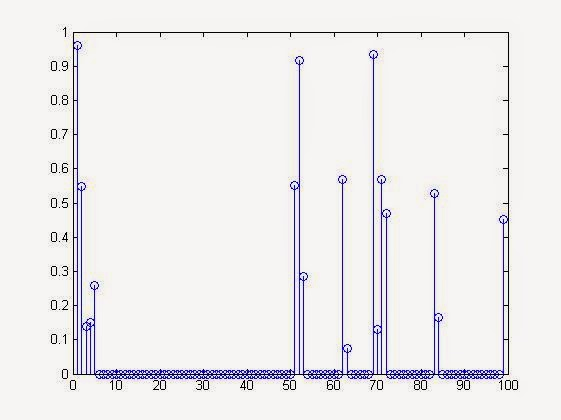Matlab Stem Plot of Block Sparse Signal Thus Generated

Comment below for solving any doubt about above implementation.

# Generate Row & column vector from Any Matrix in Matlab

Converting from a matrix to its equivalent row or column vector is very easy in MATLAB. This is particularly useful in communication where the data or here matrix has to be send serially. The matrix will be converted to a n x 1 or 1 x n vector & sent element by element. This process is often called vectorization. It has many applications in Sparse Signal Recovery & image processing also.

Suppose you have a random 5 x 5 matrix generated by rand(5,5); function  stored in variable 'x'.
In our case it is,
>> x=rand(5,5)

>> x=
0.3197    0.1639    0.6798    0.0195    0.9360
0.6711    0.4944    0.3285    0.6075    0.4886
0.5240    0.7647    0.5383    0.5388    0.6976
0.6078    0.3008    0.8378    0.2263    0.5166
0.7603    0.8389    0.2430    0.9199    0.2852

Now to convert the matrix 'x' to a column vector we just need one command.
i.e.,
>> y=x(:) %suppose we store the resultant column vector in variable named 'y'
That will throw the result,
>> y =
0.3197
0.6711
0.5240
0.6078
0.7603
0.1639
0.4944
0.7647
0.3008
0.8389
0.6798
0.3285
0.5383
0.8378
0.2430
0.0195
0.6075
0.5388
0.2263
0.9199
0.9360
0.4886
0.6976
0.5166
0.2852

& >> size(y)=  [25   1] %column vector

Now to convert the matrix 'x' to a row vector we need only slight modification of previous command command.
i.e.,
>> z=x(:)' %suppose we store the resultant column vector in variable named 'z'

That will throw the result,
>>  z =
Columns 1 through 9
0.3197    0.6711    0.5240    0.6078    0.7603    0.1639    0.4944    0.7647    0.3008

Columns 10 through 18
0.8389    0.6798    0.3285    0.5383    0.8378    0.2430    0.0195    0.6075    0.5388

Columns 19 through 25
0.2263    0.9199    0.9360    0.4886    0.6976    0.5166    0.2852

& >> size(z)=  [1    25] %row vector

NOTE: It might be an important point to note that MATLAB while vectorization (or while converting matrix to a row or column vector) reads the elements column wise not row wise, which we usually learn in our schools. For doing that in row wise ways you must take first the transpose of the original matrix & then perform above operations.

i.e.,
x=rand(5,5)
b=x'; %taking transpose to convert columns into rows & storing in a new variable 'b'

y=b(:); %Column vector when read column wise of original 'x' & stored in 'y'

z=b(:)'; %Column vector when read column wise of original 'x' & stored in 'z'

# Generate a Random Sparse Signal Vector Using Randperm Function in MATLAB

Yes Matlab does have an inbuilt sparse data type & related function, which can be used very easily to generate a random sparse Vector. ( See :Creating & Using Sparse Matrix & Vectors in MATLAB - Concepts & Examples )
But there are other ways also in which you can generate it. One of them is through randperm() function of matlab.
To generate in this way it involves a little trick & some understanding upon how the Matlab's variable/vector index works & how randperm function works.

MATLAB Code for Random Sparse Signal Vector Using Randperm Function Will look Like this:

clc
clear all
close all
n=100;  %generation 100x1 vector sparse
k=10;
%Out of 'n' elements 'k' are non zero
xorg=zeros(n,1);
%initialization of the sparse vector as a zero vector 'xorg'
idx=randperm(n);  %we are calculating the index of first 'k' non zero elements through this step
xorg(idx(1:k))=rand(k,1); %take first 'k' elements of 'idx' & fill those index with a random value
stem(xorg) %plot the sparse vector thus generated

NOTE: # initializing by xorg=zeros(n,1); is necessary since if you are not doing this, you will generate x(org) in each run, with a different dimension.
# instead of rand(k,1) one can use randn(k,1) also.

Sample Run Of the Above MATLAB's Code: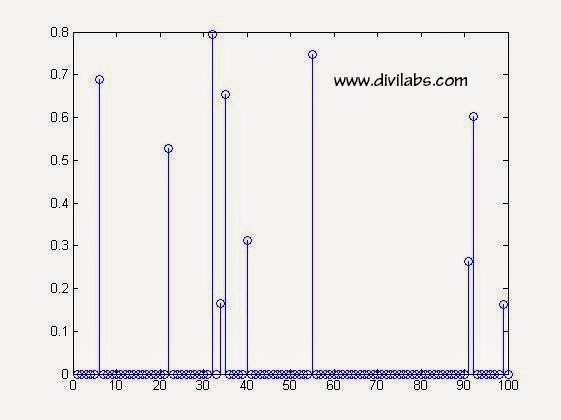Random Sparse Signal Vector Using Randperm Function in MATLAB
How the Code Works?

** xorg=zeros(n,1); -> This command generate a 'n x 1' column vector of all elements being zero.

** idx=randperm(n); -> This command will generate a '1 x n' vector in which the elements are distributed randomly between 1 to n  (including this also). There is no repetition of any number between 1 to n, i.e., every element will be unique in this vector.

e.g., in the above it is,
idx = 99    32    40    22    34    92    91    35     6    55     3    96    68    16    69 & so on

** xorg(idx(1:k))=rand(k,1); -> idx(1:k) will select first 'k' elements from 'idx' here in this example it will be  99    32    40    22    34    92    91    35     6    55 & take them to select those index of 'xorg'. Then set those index value of 'xorg' to some random generated value ranging from 0 to 1 (since we are using rand( ) function & using  stem(xorg); -> plot the generated sparse signal

You can verify that index number 99    32    40    22    34    92    91    35     6    55 are non zero & others are all zero.
In our execution, the result was.
xorg=

0
0
0
0
0
0.689214503140008
% 6th index
0
0
0
0
0
0
0
0
0
0
0
0
0
0
0
0.528533135506213
% 22nd index
0
0
0
0
0
0
0
0
0
0.794284540683907
%32nd index
0
0.165648729499781
%34th index
0.654079098476782 %35th index
0
0
0
0
0.311215042044805
%40th index
0
0
0
0
0
0
0
0
0
0
0
0
0
0
0.748151592823710
%55th index
0
0
0
0
0
0
0
0
0
0
0
0
0
0
0
0
0
0
0
0
0
0
0
0
0
0
0
0
0
0
0
0
0
0
0
0.262971284540144
%91th index
0.601981941401637
%92nd index
0
0
0
0
0
0
0.162182308193243
%99th index
0

# Calculating & visualizing the Perceived Brightness of a Color Image (RGB) in MATLAB

Calculating the Perceived Brightness of a Color Image (RGB) in MATLAB  or in simple words,
calculating the brightness of an RGB color image.

Before implementing it in matlab, we must know the conversion formula,

RGB -> Luma conversion formula. (more weight-age to green since our eyes are more sensitive to green color.)

Photometric/digital ITU-R:
(Here `0.2126`+`0.7152+``0.0722=1) `
`(Source: en.wikipedia.org/wiki/Relative_luminance)`
`Y1 = 0.2126*R + 0.7152*G + 0.0722*B`
`` ``
Digital CCIR601 (gives more weight to the R and B components):
(Here `0.299`+`0.587+``0.114=1)`
`(Source: www.w3.org/TR/AERT) `
``Y2 = 0.299*R + 0.587*G + 0.114*B``
`` ``
If ready to trade accuracy with performance, there are two approximation formulas above two:
(Source : Franci Penov answer )
``Y1a = 0.33*R + 0.5*G + 0.16*B ``
````or ````Y1a = (R+R+B+G+G+G)/6

Y2a = 0.375*R + 0.5*G + 0.125*B ```` ````
``or ``Y2a = (R+R+R+B+G+G+G+G)/8``
`` ``

Our Test Image is an RGB image: 225x609 in sizeOur Test Image is an RGB image 225x609 in size

Suppose we have read the above image through imread( ) function & stored it in a variable named 'x' .
>> x=imread('image.jpg'); %assuming that this image lies in working directory & named "image"

now size(x) will give result as,
ans =   225   609     3

NOTE: The third element displaying a value of '3' denotes the presence of 3 planes of 225x609 matrix. 1st of which the red (R) plane (designated by value 1 in the 3rd index of x), 2nd is the green(G) plane (designated by value 2 in the 3rd index of x) & 3rd is the blue (B) plane (designated by value 3 in the 3rd index of x) .

In order to access three color planes separately, type your formula like this x(:,:,1) or x(:,:,2) or x(:,:,3). See more about this at: Using MATLAB, Extracting individual Red, Blue & Green (RGB) planes, From a RGB Image, With Code

Now how to apply above three formulas?

`Applying Y1 = 0.2126*R + 0.7152*G + 0.0722*B in MATLAB our command will look like,`

`>> Y1=0.2126*x(:,:,1)+0.7152*x(:,:,2)+0.7152*x(:,:,3);`
`%to access a particular pixel luma/brightness value calculated by above formula use the command as,`

`>>Y1(200,200)`
`ans =  116 `

`%thus perceived brightness at (200,200)pixel location is 166 in a scale of 255.`

`% Now since its also stored as an image we can visualize the Brightness values of the image as,`

`>>imshow(Y1)`

The above 2 lines of code will result in another image having a single luma plane, which look like this.Perceived Brightness of a Color Image (RGB) in MATLAB  by Formula 1

`Applying Y2 = 0.299*R + 0.587*G + 0.114*B in MATLAB our command will look like,`

`>> Y2=``0.299*x(:,:,1)+0.587*x(:,:,2)+0.114*x(:,:,3);`
`>>imshow(Y2) % to see visualize the brightness `
```Perceived Brightness of a Color Image (RGB) in MATLAB  by Formula 2 ```
The above image came to be more darker than Y1 case, due to the fact more weight-age is distributed among R & B value than G.